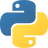Make use of a qualified tutor to get the answer

Q:

# ** python

``````#** is the exponent symbol in Python, so:
print(2 ** 3)
#output: 8``````
4
``````quotient = 3 * 4
1
``//	'a' // 'b'	(Floor Division) Quotient when 'a' is divided by 'b', rounded to the next smallest whole number``
5
``````# ** means modulus. or exponent
x = 6
y = 2
print(x**y) # will print 6 raised to power 2``````
0
``````When it iterating over a dictionary you are only able to iterate over
the keys not the values. The ** when placed before a variable will allow
you to iterate and unpack both key and value pairs. Because you are
unpacking both key and value this will return the result as a dictionary.``````
0